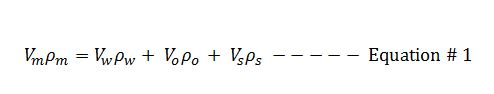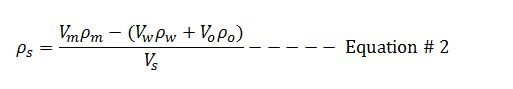## Solid Density From Retort Analysis Mud Calculation

Retort analysis is the method to determine solid and liquid components in the drilling fluid. In this article, we will adapt mass balance and retort analysis data to determine solid density in the mud.Mass balance for mud is listed below;We can rearrange the Euqation#1 in order to determine the solid density## Decrease oil/water ratio

Decrease oil/water ratio: The concept of decrease oil water ratio is to increase water volume in the system without any changes in oil volume to meet new oil water ratio.

How can we decrease oil water ratio to 70/30?

Let’s make it simple so I still use the same information as my previous post. We start with 100 bbl of mud and we have the following information from the retort analysis.

Retort analysis:

% by volume oil = 56

% by volume water = 14

% by volume solids = 30

According to this retort analysis, the oil water ratio is 80/20 (learn how to calculate oil water ratio from a retort analysis) and there are 56 bbl of oil, 14 bbl of water and 30 bbl of solid from 100 bbl of mud.

In order to decrease oil water ratio, water must be added but oil volume remains the same. Therefore, 56 bbl of oil will represent 70% of oil ratio for the new system. We give X equals to the new total liquid volume (combination of oil and water volume).

Then; 70 = (56×100) ÷X

X = 80.0 bbl

Total liquid volume is equal to 80.0 bbl.

Oil volume is still the same but water volume will be added into the system. With this concept, the volume of water will added into the system can be described with the following relationship;

Water added = new total liquid volume – original volume

Water added = 80 – 70 = 10 bbl

If you have the total mud volume of 300 bbl, you will need 30 bbl of water added (10 x 300 ÷ 100) in order to decrease oil water ratio from 80/20 to 70/30

## Increase oil/water ratio

The concept of increase oil water ratio is to increase oil volume in the system without any changes in water to meet new oil water ratio.

How can we increase oil water ratio from 80/20 to 85/15?

Let’s make it simple to understand. We start with 100 bbl of mud and we have the following information from the retort analysis.

Retort analysis:

% by volume oil = 56

% by volume water = 14

% by volume solids = 30

According to this retort analysis (learn how to calculate oil water ratio), the oil water ratio is 80/20 and there are 56 bbl of oil, 14 bbl of water and 30 bbl of solid from 100 bbl of mud. In order to increase oil water ratio, oil must be added and water volume remains the same. Therefore, 14 bbl of water will represent only 15% of water ratio for the new system. We give X equals to the new total liquid volume.

Then; 15 = (14×100)÷X

X = 93.33 bbl

Total new liquid volume is 93.33 bbl

Barrel of base oil added per 100 bbl of mud

Oil added = new total liquid volume – original volume

Oil added = 93.33 – 70 = 23.33 bbl

It means that you need to add oil 23.33 bbl per 100 bbl of original mud without adding any volume of water in order to achieve 85/15 oil water ratio.

Please find the Excel sheet for calculating how to increase oil water  ratio.

## Calculate Oil-Water Ratio from Retort DataOil Water Ratio (OWR) is a figure representing the fraction of oil and water in oil based drilling mud.  Generally speaking, it is a ratio between the percent oil in liquid phase and the percent water in liquid phase. In order to determine OWR, volume of oil/water/solid in drilling mud comes from a retort analysis. A sample of oil based mud is controlled burnt in a retort kit at required temperature. When the mud is heated, water and oil will be extracted out and solid is left in the retort kit. The retort analysis report shows percentage of each component by volume so we use data from the retort analysis to determine oil water ratio.

The formulas below demonstrate how to calculate oil water ratio from retort data.

a) % oil in liquid phase = (% by volume oil x 100) ÷ (% by volume oil + % by volume water)

b) % water in liquid phase = (% by volume water x 100) ÷ (% by volume oil + % by volume water)

c) Result: The oil/water ratio equals to the percent oil in liquid phase and the percent water in liquid phase.

Example: Determine oil water ratio from following information

Data from a retort analysis:

% by volume oil = 56

% by volume water = 14

% by volume solids = 30

Solution:

a) % oil in liquid phase = (56 x 100) ÷ (56+14)

% oil in liquid phase = 80

b) % water in liquid phase = (14 x 100) ÷ (56+14)

% water in liquid phase = 20

c) According to this retort report, the oil/water ratio equals to 80/20.

Ref books: Lapeyrouse, N.J., 2002. Formulas and calculations for drilling, production and workover, Boston: Gulf Professional publishing.

Bourgoyne, A.J.T., Chenevert , M.E. & Millheim, K.K., 1986. SPE Textbook Series, Volume 2: Applied Drilling Engineering, Society of Petroleum Engineers.

Mitchell, R.F., Miska, S. & Aadny, B.S., 2011. Fundamentals of drilling engineering, Richardson, TX: Society of Petroleum Engineers.

## Determine the density of oil and water mixture

You normally get the oil/water ratio from a retort so you can use these numbers to determine density oil and water mixture by the following formula.

(V1)(D1) + (V2)(D2) = (V1 + V2)DF

Where;

V1 = percentage of oil

V2 = percentage of water

D1 = density of oil

D2 = density of water

DF = final density of mixed fluid between oil and water

Example: If the oil/water (o/w) ratio is 80/20 (80% oil and 20% water), please determine the density of mixed fluid between oil and water from the retort. Oil density = 7.0 ppg. Water density = 8.33 ppg.

V1 = 0.8

V2 = 0.2

D1 = 7.0

D2 = 8.33

(V1)(D1) + (V2)(D2) = (V1 + V2)DF

(0.8)(7.0) + (0.2)(8.33) = (0.8 + 0.2) DF

5.60 + 1.67= 1.0 DF

7.27 = DF

The density of the oil/water mixture = 7.27 ppg

Please the Excel sheet used to calculate the density of oil and water mixture.